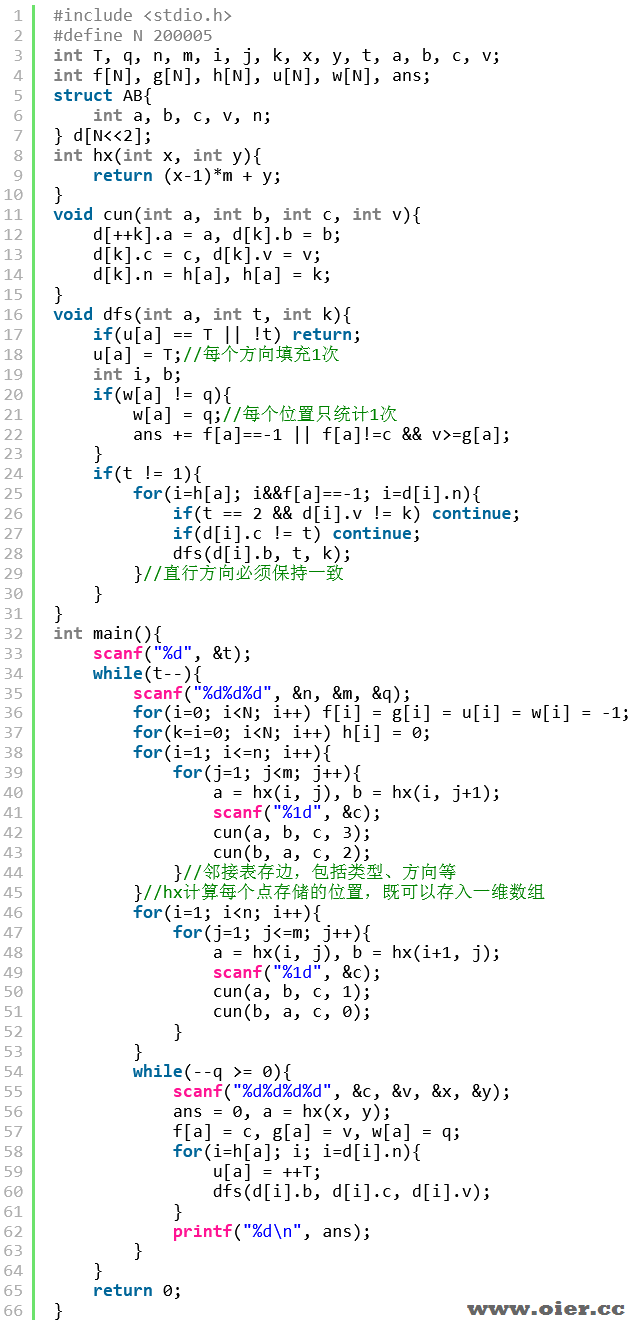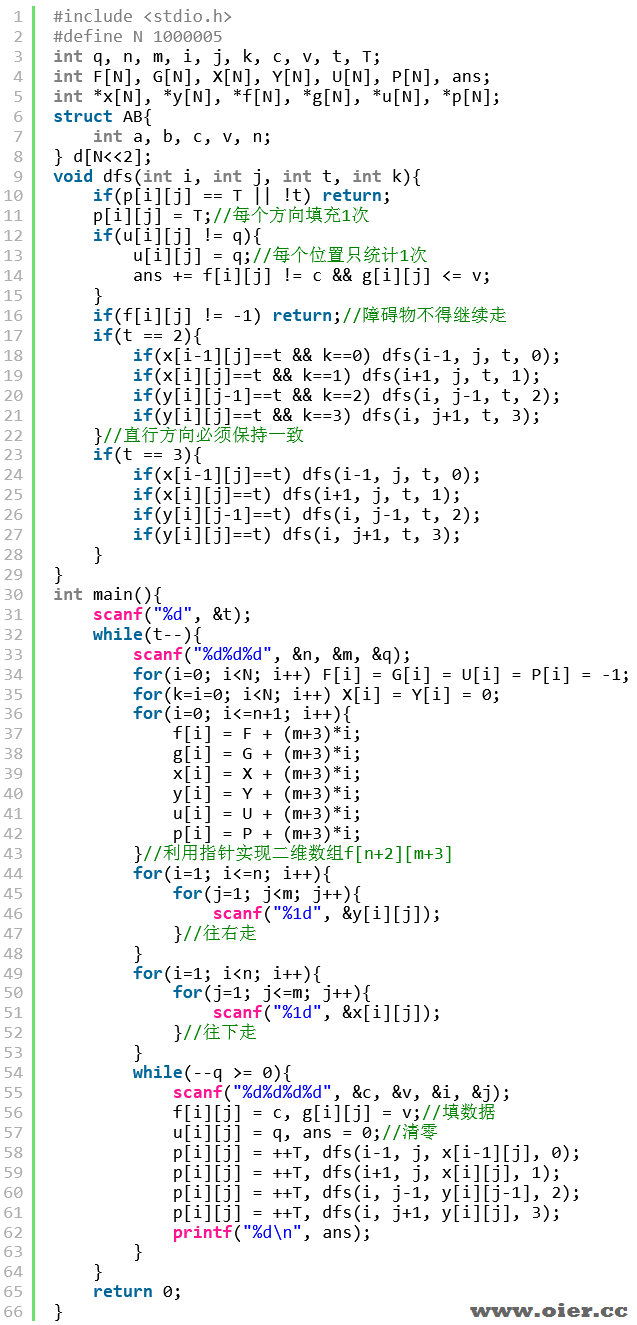P7963棋局（NOIP2021）
83+

题目背景

“既然我们都会玩但只能玩一点点，不如我们自己搞个缝合怪出来吧！”

题目描述

– 对于任意 $i=0,1,\ldots,k-1$，坐标 $(x_i,y_i)$ 和 $(x_{i+1},y_{i+1})$ 之间必须有网格线直接相连，也就是说**走子必须沿着网格线走**；
– 对于任意 $i\not=j$，必须有 $(x_i,y_i)\not=(x_j,y_j)$，也就是说走子过程中不能经过重复位置，特别地 $(x_0,y_0)\not=(x_k,y_k)$，也就是说**不能原地不动（或走回原地）**；
– 对于任意 $i=1,\ldots,k-1$，坐标 $(x_i,y_i)$ 上必须没有棋子，也就是说**走子时不能越过已有的棋子**；
– 若 $(x_k,y_k)$ 上没有棋子，称为普通走子，否则称为吃子。在吃子过程中，设正在走的棋子颜色为 $\mathit{col}_1$，等级为 $\mathit{lv}_1$，被吃的棋子颜色为 $\mathit{col}_2$，等级为 $\mathit{lv}_2$，则必须满足 $\mathit{col}_1\not=\mathit{col}_2,\mathit{lv}_1\geq\mathit{lv}_2$，换句话说**只能吃与自己颜色不同，且等级不高于自己等级的棋子**。

– 如果 $\mathit{opt}_i=0$，代表此路不通，走子时不能经过这条网格线；
– 如果 $\mathit{opt}_i=1$，代表这条网格线是一条“普通道路”，每次走子时棋子最多只能经过 $1$ 条普通道路。
– 如果 $\mathit{opt}_i=2$，代表这条网格线是一条“直行道路”，每次走子时棋子可以经过任意条直行道路，但只能**一直沿横向或一直沿纵向走，不能转弯**。如沿直行道路从 $(1,1)$ 经过 $(1,2)$ 走到 $(1,3)$ 是可以的，但是从 $(1,1)$ 经过 $(1,2)$ 走到 $(2,2)$ 不行。
– 如果 $\mathit{opt}_i=3$，代表这条网格线是一条“互通道路”，每次走子时棋子可以经过任意条互通道路，且中途可任意转弯。

1
3 3 5
13
22
23
010
233
0 1 2 3
1 2 2 1
1 3 1 2
0 2 3 2
1 3 2 2

4
3
3
3
2

2
2 3 4
22
33
123
0 2 1 2
0 1 2 1
1 2 1 3
0 3 2 2
3 2 3
3
1
3
32
32
0 2 1 2
1 2 3 2
0 1 2 2

3
4
4
2
5
5
1

说明

**【样例解释 #1】**

**【数据范围】**

| 测试点编号 | $n \times m \le$ | $q \le$ | 特殊性质 |
|:-:|:-:|:-:|:-:|
| $1 \sim 2$ | $100$ | $50$ | 无 |
| $3 \sim 6$ | $5000$ | $2000$ | 无 |
| $7 \sim 8$ | $2 \times {10}^5$ | ${10}^5$ | 不存在“直行道路”与“互通道路” |
| $9 \sim 11$ | $2 \times {10}^5$ | ${10}^5$ | 不存在“互通道路” |
| $12 \sim 14$ | $2 \times {10}^5$ | ${10}^5$ | 不存在“直行道路” |
| $15 \sim 16$ | $2 \times {10}^5$ | ${10}^5$ | $\mathit{lv}_i = i$ |
| $17 \sim 18$ | $2 \times {10}^5$ | ${10}^5$ | $\mathit{lv}_i = q – i + 1$ |
| $19 \sim 21$ | $2 \times {10}^5$ | $2000$ | $n, m \le 1000$ |
| $22 \sim 25$ | $2 \times {10}^5$ | ${10}^5$ | 无 |